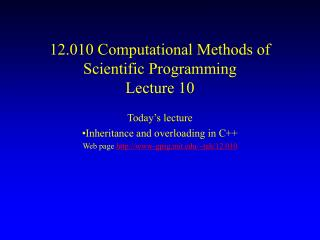# 12.010 Computational Methods of Scientific Programming Lecture 10 - PowerPoint PPT PresentationDownload Presentation12.010 Computational Methods of Scientific Programming Lecture 10

12.010 Computational Methods of Scientific Programming Lecture 10
Download Presentation## 12.010 Computational Methods of Scientific Programming Lecture 10

- - - - - - - - - - - - - - - - - - - - - - - - - - - E N D - - - - - - - - - - - - - - - - - - - - - - - - - - -
##### Presentation Transcript

1. 12.010 Computational Methods of Scientific ProgrammingLecture 10 Today’s lecture Inheritance and overloading in C++ Web page http://www-gpsg.mit.edu/~tah/12.010

2. Previous Lecture • Basic features of C++ • Adds formal class concept to C, making it object-oriented • Class is like a derived type except • It is better encapsulated • It has “methods” • Invoking a method is like sending a message to the object, object contains its own logic saying what to do. • E.g the String class String s1; s1.set(“Hello”); printf(“%s\n”,s1.s()); 12.010

3. Inheritance Want new class uString. Like String except that the strings will be converted and stored in upper case. e.g. String uString set() s() set() s() uString s; s.set(“Hello”); printf(“%s\n”,s.s()); HELLO String s; s.set(“Hello”); printf(“%s\n”,s.s()); Hello 12.010

4. uString extends String • No need to write uString from scratch. • Inherit most code from String. • Extend String::set to capitalise. • A uString is a String with some extra feature. String Base class set() s() uString Derived class 12.010

5. C++ InheritanceExample • New interface for uString /* Extend String class to uString */ /* uString stores strings as upper case */ class uString : public String { public: void set( char *); /* Set a uString */ }; 12.010

6. uString set method /* Set str to point to a private copy of s */ void uString::set(char *s) { int i; String::set(s); for (i=0;i<strlen(s);++i) { if ( str[i] >= 'a' && str[i] <= 'z' ) { str[i] = toupper(str[i]); } } } Base class method “protected” (not “private”) 12.010

7. uString in action! main() { String s1; uString s2; printf("Executable code starting\n"); s1.set("Hello"); printf("%s\n",s1.s()); s2.set("Hello"); printf("%s\n",s2.s()); printf("Executable code ending\n"); } 12.010

8. Overloading Can redefine operators e.g. + to operate on classes e.g. coord =() +() coord p1, p2, p3; p3 = p1 + p2 This would then do if p1=p2=(1,1,1) p3 = (2,2,2) 12.010

9. Overloading Have to define the meaning of + and = for a coord class object. Language defines meaning for integer, float, double etc but now we can define extra meanings. coord coord::operator+ (coord c2) { coord temp; temp.cx = cx + c2.cx; temp.cy = cy + c2.cy; temp.cz = cz + c2.cz; return(temp); } class coord{ public: coord operator+(coord ); private: int cx; int cy; int cz; }; 12.010

10. Homework • http://mitgcm.org/~cnh/12.010/Lec10/homework3.html • Due Oct 27th • E-mail homework and question to cnh@mit.edu 12.010